Question

# Where in it's motion is the velocity zero for a spring oscillating up and down with...

1. Where in it's motion is the velocity zero for a spring oscillating up and down with simple harmonic motion?

 Top Bottom Middle Top and Bottom

Top and bottom.

Velocity is zero at extreme points.

Velocity is maximum at middle point.

#### Earn Coins

Coins can be redeemed for fabulous gifts.

Similar Homework Help Questions
• ### A mass is oscillating up and down at the end of a spring. A graph of...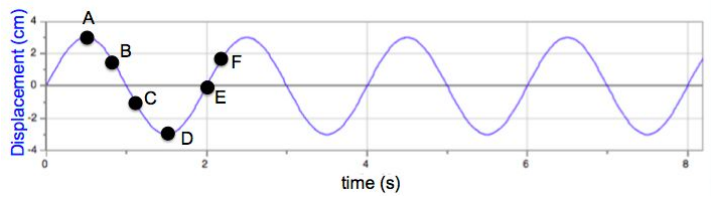A mass is oscillating up and down at the end of a spring. A graph of displacement as a function of time is shown where up is the positive direction. a) What is the period of this motion? Explain b) At which point or points is the acceleration positive? Explain c) At which point or points does the mass have a zero velocity but nonzero net force? Explain d) At which point or points is the net force on the...

• ### A mass is oscillating up and down at the end of a spring similar to Activity...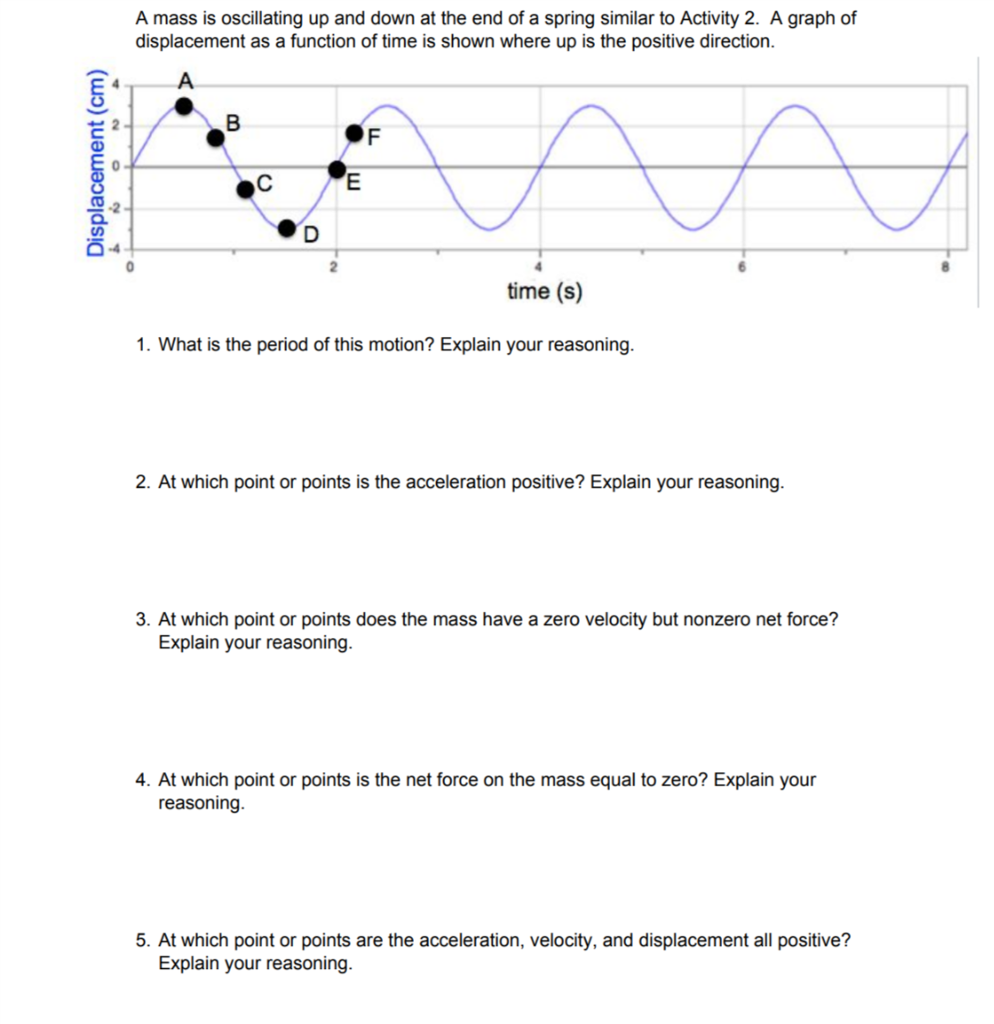A mass is oscillating up and down at the end of a spring similar to Activity 2. A graph of displacement as a function of time is shown where up is the positive direction O-4 time (s) 1. What is the period of this motion? Explain your reasoning. 2. At which point or points is the acceleration positive? Explain your reasoning. 3. At which point or points does the mass have a zero velocity but nonzero net force? Explain your...

• ### A mass is attached to a spring & is oscillating up & down. The position of...

A mass is attached to a spring & is oscillating up & down. The position of the oscillating mass is given by... y=(3.2 cm)*Cos[2*3.14*t/(0.58 sec)]; where t is time. Determine (a) the period of this motion; (b) the first time the mass is at position y=0. Please show all work.

• ### which is not an example of simple harmonic motion a mass attached to a spring oscillating...

which is not an example of simple harmonic motion a mass attached to a spring oscillating on frictonless table a stone dropped in a pond an obj in uniform circular motion a pendulum

• ### If a 10g mass is oscillating in simple harmonic motion with a spring constant of 2N/m,...

If a 10g mass is oscillating in simple harmonic motion with a spring constant of 2N/m, find the time for one full oscillation (period).

• ### If a 10 g mass is oscillating in a simple harmonic motion with a spring constant...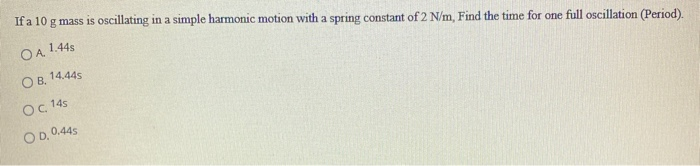If a 10 g mass is oscillating in a simple harmonic motion with a spring constant of 2 N/m, Find the time for one full oscillation (Period). ОА, 1.44s 14.445 OB. Oc 145 OD. 0.445

• ### A mass attached to a spring is moving up and down in simple harmonic motion. The...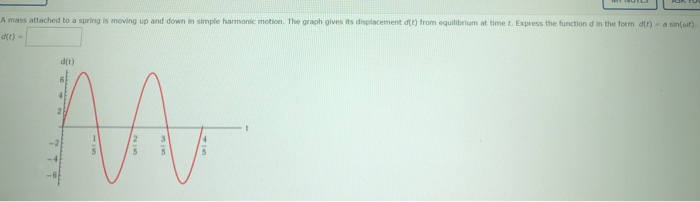A mass attached to a spring is moving up and down in simple harmonic motion. The graph gives its displacement (t) from equilibrium at time. Express the function in the form (t) - a sin(at). d(t) 2 1 2 3 5 5 5

• ### An object of mass m is attached to a spring and it is oscillating back and...

An object of mass m is attached to a spring and it is oscillating back and forth in simple harmonic motion. What is the average momentum of the mass over many oscillations?

• ### Explain why the displacement of a weight moving up and down on a spring can be...

Explain why the displacement of a weight moving up and down on a spring can be considered a form of simple harmonic motion that can be explained using a wave equation of the form y = a sin (bt + c) where t is the time. Explain the interpretation of y, a, b, c in this context.

• ### . Simple Harmonic Motion: An object is attached to a coiled spring. It is pulled down a distance of 6 inches from its equilibrium position and released. The period of the motion is 4 seconds. a....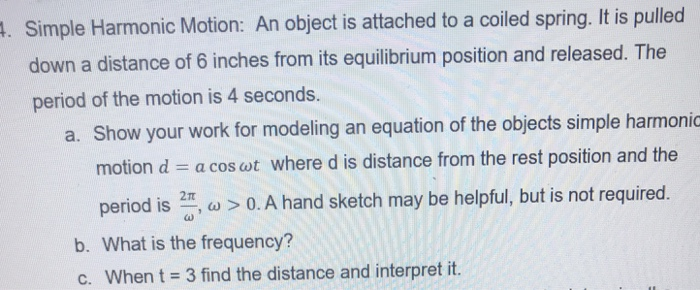. Simple Harmonic Motion: An object is attached to a coiled spring. It is pulled down a distance of 6 inches from its equilibrium position and released. The period of the motion is 4 seconds. a. Show your work for modeling an equation of the objects simple harmonic motion d a cos wt where d is distance from the rest position and the 0. A hand sketch may be helpful, but is not required. period is b. What is the...